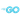# Indexing Strings and Runes in Go

I've been using exercism's Go track to help me learn Go. It's been an amazing experience so far. Exercism is mentor-based exercise platform that allows students to request feedback from mentors.

After working on a Go exercise that involves comparing the difference "distance" between two strings, John Arundel (who is also a Go maintainer) pointed out in my mentor review, that I need to consider the implications of indexing a string in Go versus indexing runes.

Here's my original implementation:

Original Solution: String Comparison
``````// Package hamming implements utilities to find the Hamming Distance
// between two strands of DNA
package hamming

import "errors"

// Distance returns the Hamming Distance between two strands of DNA
func Distance(a, b string) (int, error) {
if len(a) != len(b) {
return 0, errors.New("the two dna strings should be equal length")
}

var distance int
for i := range a {
if a[i] != b[i] {
distance++
}
}

return distance, nil
}``````

This solution would return a hamming distance value of `1` for the given string inputs `"aaa"` and `"aua"`.

However, consider the case of the string inputs `"aua"` and `"aüa"`. This implementation would actually error out since the character `"ü"` is represented by two bytes.

``Error: the two dna strings should be equal length``

## Strings in Go are a slice of arbitrary bytes

In Go, strings are actually a slice of arbitrary bytes and do not necessarily hold Unicode or UTF-8 text.

When we store a character value in a string, we store its byte-at-a-time representation, not the actual character representation.

So, when indexing a string, the index accesses the individual bytes in the string, not its characters, since a string is actually a slice of bytes.

With this in mind, when we iterate over a string, the index doesn't move by each single UTF-8 encoded character increments, but instead by every byte.

If we wish to increment the index by character, we must either cas the slice to a string or rune.

For example, consider the characters `"u"`, and `"ü"`:

``````const str = `ü`

fmt.Printf("hex bytes: ")
for i := 0; i < len(str); i++ {
fmt.Printf("%x ", str[i])
}
fmt.Printf("\n")``````
``````plain string: ü
ASCII-only quoted string: "\u00fc"
individual bytes (in hexadecimal): c3 bc``````

What this means is that the character `"ü"` is represented by:

• The bytes (in UTF-8 encoding): `c3 bc`
• The hexadecimal value: `00fc`

And likewise the character `"u"` is represented by:

``````const str = `u`

fmt.Printf("plain string: %s\n", str)

fmt.Printf("quoted string: %+q\n", str)

fmt.Printf("hex bytes: ")
for i := 0; i < len(str); i++ {
fmt.Printf("%x ", str[i])
}
fmt.Printf("\n")``````
``````plain string: u
ASCII-only quoted string: "u"

## Runes in Go represent "characters"

In other programming languages, the "characters" in strings are sometimes referred to as "code points". In Go, the term used is a "rune". For example, the character `"ü"` is represented by: the code point U+00fc (with the hexadecimal value 00fc)

Runes in Go are Unicode characters, which can be up to 4 bytes in size.

So, if we are interested in a string as runes, we should convert the string to a slice of runes:

``ar, br := []rune(a), []rune(b)``

This allows us to safely compare the runes:

• The lengths of the rune slices
• Iterate over one of them, and compare each rune with the corresponding rune in the other.
Rune Comparison Solution
``````// Package hamming implements utilities to find the Hamming Distance
// between two strands of DNA
package hamming

import "errors"

// Distance returns the Hamming Distance between two strands of DNA
func Distance(a, b string) (int, error) {
ar, br := []rune(a), []rune(b)
^^^^^^^^^^^^^^^^^^^^^^^^^^^^^^
Convert the strings to a slice of runes

if len(ar) != len(br) {
return 0, errors.New("the two dna strings should be equal length")
}

var distance int
for i := range ar {
if ar[i] != br[i] {
distance++
}
}

return distance, nil
}``````